﻿ Graph - 演算法筆記

Graph Property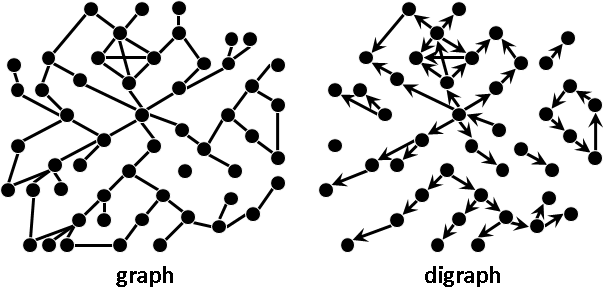Degree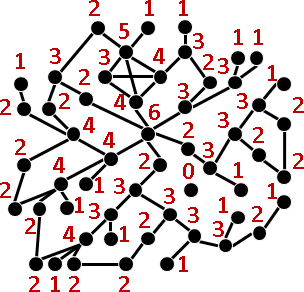Neighbor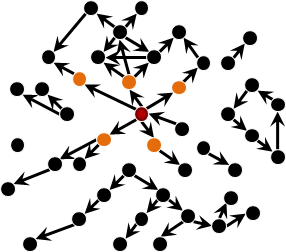```adjacent：相鄰、鄰接。只走一步，可以抵達。
connected：相連、連通。不限步數，可以抵達。
```

Distance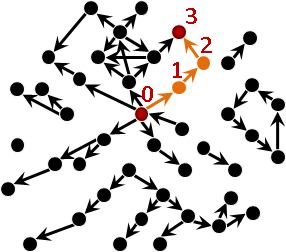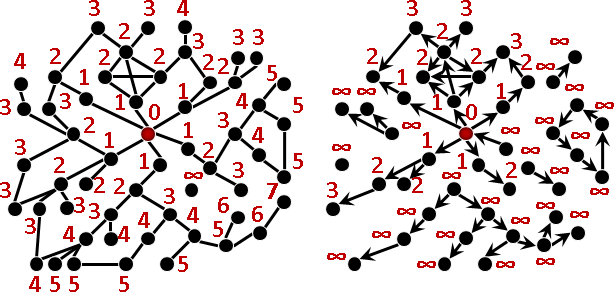```eccentricity：偏心距。從一個點出發的最長距離。
diameter：直徑。一張圖（的每個起點之中）最長的偏心距。一張圖最長的距離。
```

Graph Operation

Intersection GraphDependency Graph【尚無正式名稱】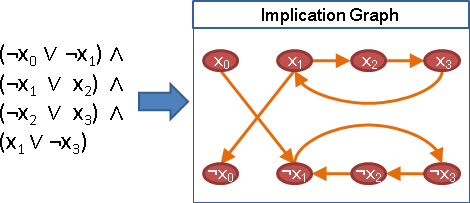UVa 10926

Subgraph / Supergraph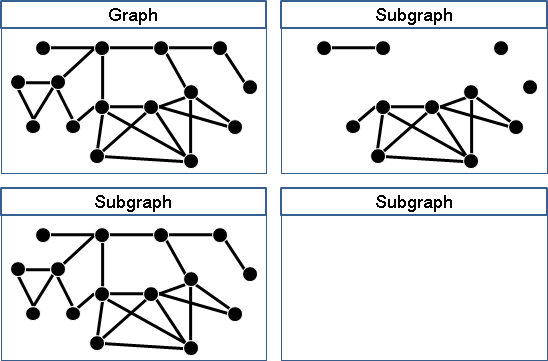subgraph和supergraph是相對的。如果A是B的子圖，那麼B就是A的父圖。我們習慣只講子圖，講一個就等於兩個都講了。

Induced Subgraph / Induced Supergraph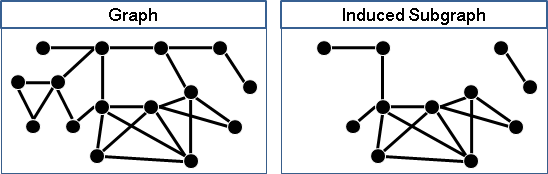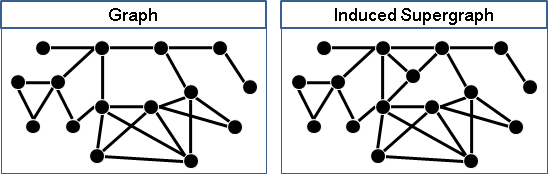induced subgraph和induced supergraph是相對的。如果A是B的導出子圖，那麼B就是A的導出父圖。我們習慣只講導出子圖，講一個就等於兩個都講了。

Minor / Subdivision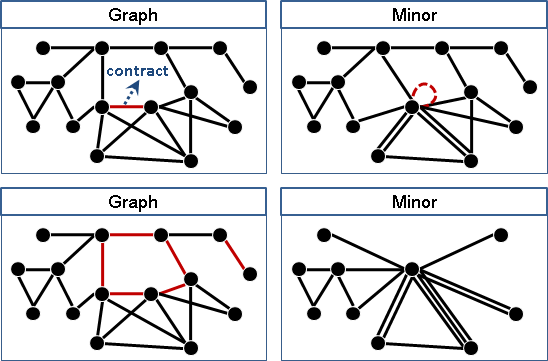minor和subdivision是相對的。一般只討論minor。

Oriented Graph / Underlying Graph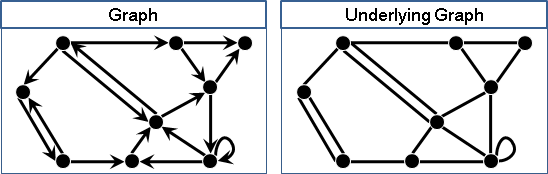Complement Graph（Complement）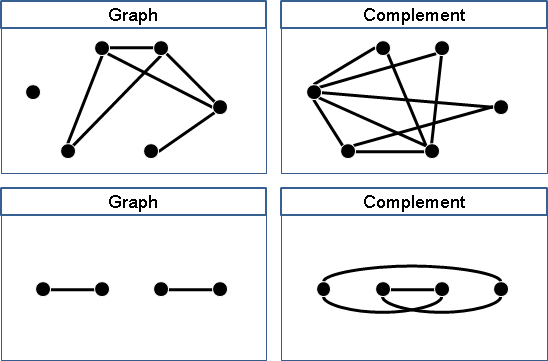Reverse Graph（Transpose）Line Graph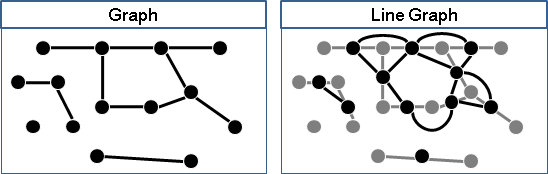UVa 10988 11175

Dual Graph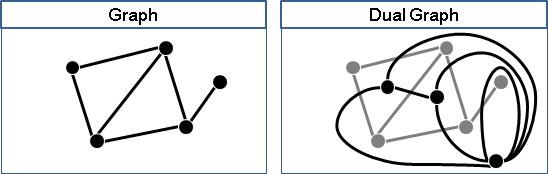Hypergraph

Hypergraph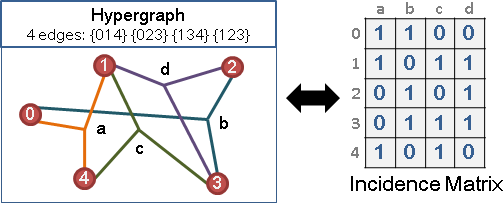「圖」是談兩個東西之間的關係，「超圖」則是談多個東西之間的關係，例如三個東西之間的關係。

Graph Theory

Graph TheoryGeometric Graph Theory

Topological Graph Theory

```minor containment problem 問一張圖是不是有某個minor。至少是NP-complete。
https://en.wikipedia.org/wiki/Graph_minor
https://en.wikipedia.org/wiki/Robertson–Seymour_theorem
https://en.wikipedia.org/wiki/Graph_structure_theorem
https://en.wikipedia.org/wiki/Graph_sandwich_problem
```

Extremal Graph Theory

Structural Graph Theory

Algebraic Graph Theory與Spectral Graph Theory